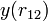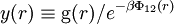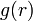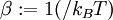Cavity correlation function

The cavity correlation function,$y(r_{12})$, is given by$y(r) \equiv {\mathrm{g}}(r) /e^{-\beta \Phi_{12}(r)}$
where$g(r)$ is the pair distribution function,$\Phi_{12}(r)$ is the pair potential, and$\beta := 1(/k_B T)$, where$k_B$ is the Boltzmann constant and$T$ is the temperature.